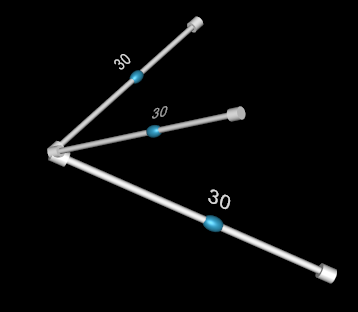# Slider rotation appears incorrect - vtkSliderRepresentation3D

I have created a small test program that creates vtkSliderWidgets with the vtkSliderRepresentation3D. I create one slider per axis (not necessarily along the main coordinate system axes). Even though the three sliders have the same start point, one of the sliders seems to start offset from the start point and pointing slightly in the wrong direction.

sliderRep->SetPoint1InWorldCoordinates(0.0, 0.0, -6.0); // Note: All sliders have the same start point
sliderRep->SetPoint2InWorldCoordinates(x, y, z);

The (x,y,z) coordinates for the axes are the following where the x-axis looks rotated incorrectly:

(0.8060793615028174, -4.596267436520017, -6.0)
(-6.465520758172843, -1.1339033066529178, -6.0)
(0.0, 0.0, 0.0)

vtkSliderRepresentation3D seems to want to rotate some geometry aligned along the x-axis with the slider axis defined by point1 and point2. Could there be an issue with the rotation calculation?

Also note that I have tried using vtkLineWidget instead of vtkSliderWidget and that seems to render the axes correctly with the correct start point and end point.

Got any ideas?

Is the problem that sliders appear incorrectly or they don’t rotate objects as you expect? Can you post a screenshot?

Here is a screenshot of the sliders - one for each axis and you will see one which does not have the same start point as the others.

Here is my test with vtkLineWidget with the same coordinates - it appears to render as expected.

Not the same starting point is a minor issue compared to the text being distorted and mirrored. You would normally use these sliders in a 2D renderer layer that does not move/rotate with your 3D scene content.

My application does use sliders a part of a scene that can be rotated. Seems this could be a normal use case scenario? Currently used for moving slices inside a volume dataset.

The start point is incorrect, but so is probably the end point since the rotation used is probably not what it should be (guess maybe the middle point could be correct though) .

I do believe correctness should be important when it comes to positioning though also agree that text should be readable preferably from any rotation. Think the position issue could easily be fixed?

My application does use sliders a part of a scene that can be rotated. Seems this could be a normal use case scenario? Currently used for moving slices inside a volume dataset.

I recently implemented something very similar but I dont get the problem with the widgets orientation:

you can check out how it’s done here, here and here.

Would it be possible for you to test with the coordinates provided above and see if the sliders appear correct for you?

point1: (0.0, 0.0, -6.0)
point2:
(0.8060793615028174, -4.596267436520017, -6.0) -> this should appear incorrect
(-6.465520758172843, -1.1339033066529178, -6.0)
(0.0, 0.0, 0.0)

I have also pushed my test code to a repo if you would like to try there.

https://bitbucket.org/jornskaa/vtksliderwidget/

is it that maybe point1 and point2 are swapped wrt the linewidget case?

``````  sliderRep->SetPoint1InWorldCoordinates(x+0.5, y, z);
sliderRep->SetPoint2InWorldCoordinates(0,0,0);
//...
auto sliderWidgetX = createSliderWidget(30, renderWindowInteractor,  1, 0, 0);
auto sliderWidgetY = createSliderWidget(30, renderWindowInteractor,  0, 1, 0);
auto sliderWidgetZ = createSliderWidget(30, renderWindowInteractor,  0, 0, 1);
``````I have tried swapping the coordinates as well, but the situation is still the same/similar. Have you tried to use the coordinates I provided? Would be interesting if you can reproduce the situation. In your post you use the main coordinate axes which I think should work fine for me as well.

I have found vtkSliderRepresentation3D::BuildRepresentation to calculate an incorrect angle of rotation of the slider mesh in some cases. I have tried correcting this by replacing:

``````vtkMath::Normalize(v);
vtkMath::Cross(v,x,axis);
double theta, axisLen = vtkMath::Norm(axis);
if ( axisLen != 0.0 )
{
theta = vtkMath::DegreesFromRadians( asin( axisLen ) );
}
``````

With this:

``````vtkMath::Normalize(v);
vtkMath::Cross(v,x,axis);
double dotProd = vtkMath::Dot(v, x);
double theta, axisLen = vtkMath::Norm(axis);
if ( axisLen != 0.0 )
{
theta = vtkMath::DegreesFromRadians(vtkMath::Pi() - atan2(axisLen, dotProd));
}
``````

Basically a directional angle is found instead of the one found by asin, and that seems to work for my case. Haven’t tested this a lot yet, but seems to work for me right now. Hopefully a similar fix can be made to VTK as well1 Like